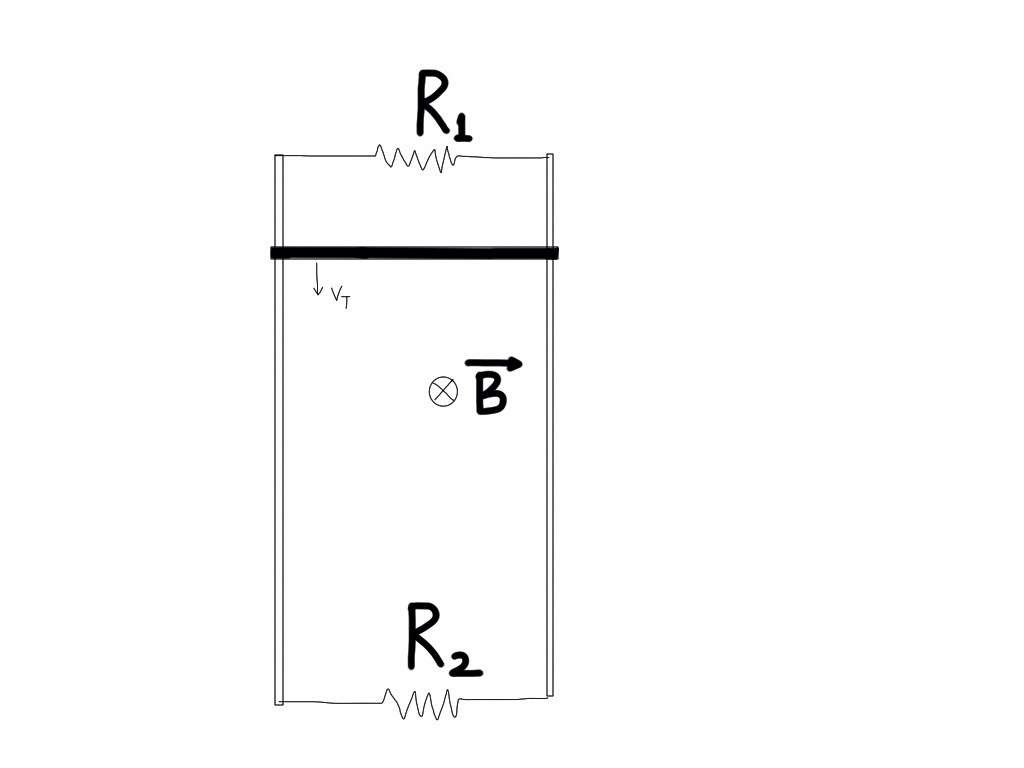# Terminal velocityA system of long, parallel conducting rails is set up in the vertical plane and joined at both the ends by resistors as shown in the figure. A uniform magnetic field exists perpendicular to the plane of the rails denoted by $\vec{B}$. A rod whose length is equal to the separation between the rails is allowed to fall freely and attain a terminal velocity. If the powers dissipated by the resistors $R_1$ and $R_2$ at steady state are $0.76 \text{ W}$ and $1.2\text{ W},$ respectively, then what is the terminal velocity of the rod?

Details and Assumptions

• The length and mass of the rod are 1 m and 0.2 kg.
• The acceleration due to gravity is $9.8\text{ m/s}^2$.
• The magnitude of the magnetic field is 0.6 T.
• The rails are long enough so that the steady state conditions can be easily analysed.
×